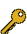Last Update: 2020-09-26 15:59:01

## 4-c. \$B>xN1(B

\$B:G=*99?7F|;~!'(B2020-09-26 15:59:01\$B\$3\$NJ,N`\$G\$h\$/;H\$o\$l(B
\$B\$F\$\$\$k%-!<%o!<%I(B
\$B%-!<%o!<%I(B\$B
Process Optimization1\$B7o(BExtractive distillation1\$B7o(BSalt effect1\$B7o(BSimplified temperature difference control (STDC)1\$B7o(Bprocess design1\$B7o(Bvapor recompressed heat pump1\$B7o(Bazeotropic mixtures1\$B7o(Bdividing-wall distillation column1\$B7o(BDividing wall distillation column (DWDC)1\$B7o(Bmembrane + distillation hibrid process1\$B7o(Bternary azeotrope1\$B7o(BSimplified double temperature difference control (SDTDC)1\$B7o(B\$B\$BHV9f(B\$B9V1iBjL\!?H/I=\$B%-!<%o!<%I(B\$BH/I=7A<0(B
148A kind of topological structures of vapor recompressed dividing-wall distillation columns
(Beijing U. Chemical Tech.) \$B!{(B(\$B3\$(B)Zang Lijing\$B!&(B (\$B3\$(B)Huang Kejin\$B!&(B (\$B3\$(B)Qian Xing\$B!&(B (\$B3\$(B)Yuan Yang\$B!&(B Zhang Liang\$B!&(B Wang Shaofeng\$B!&(B Chen Haisheng
dividing-wall distillation column
vapor recompressed heat pump
process design
O
208Controllability comparison of the four-product Petlyuk dividing wall distillation column using temperature control schemes
(Beijing U. Chemical Tech.) \$B!{(B(\$B3\$(B)Qian Xing\$B!&(B (\$B3\$(B)Huang Kejin\$B!&(B Chen Haisheng\$B!&(B (\$B3\$(B)Yuan Yang\$B!&(B Zhang Liang\$B!&(B Wang Shaofeng
Dividing wall distillation column (DWDC)
Simplified temperature difference control (STDC)
Simplified double temperature difference control (SDTDC)
O
277\$B6&J(:.9gJ*J,N%\$rxN1(B+\$BKlJ#9g%W%m%;%9\$N9=@.(B
(\$BL>9)Bg1!9)(B) \$B!{(B(\$B3X(B)\$BC]Fb(B \$BWB(B\$B!&(B (\$BL>9)Bg(B) (\$B@5(B)\$B4dED(B \$B=\$0l(B\$B!&(B (\$B@5(B)\$BFn1@(B \$BN<(B\$B!&(B (\$B@5(B)\$B?9(B \$B=(
membrane + distillation hibrid process
azeotropic mixtures
ternary azeotrope
P
655\$B1v\$K\$h\$k8z2L\$rMQ\$\$\$?%(%?%N!<%k@:@=%W%m%;%9\$N3+H/(B
(\$B5~Bg9)(B) \$B!{(B(\$B3X(B·\$B5;4p(B)\$BCfB<(B \$BM'5*(B\$B!&(B (\$B3\$(B)\$B\$"\$k\$+\$s\$?\$i\$"\$S\$i(B \$B\$X\$9!<\$9\$i\$U\$!\$(\$k(B\$B!&(B (\$BFAEgBg
Extractive distillation
Salt effect
Process Optimization
P

\$B9V1i?=\$79~\$_0lMw(B(\$B%7%s%]%8%&%`HV9f!&9V1iJ,N`HV9fJL(B)

\$B
\$B2=3X9)3X2q(B \$BBh(B85\$BG/2q(B